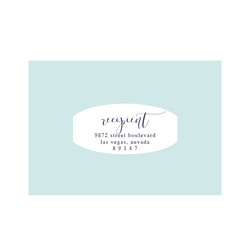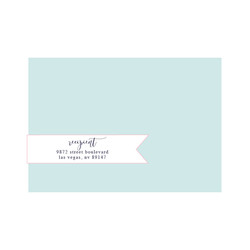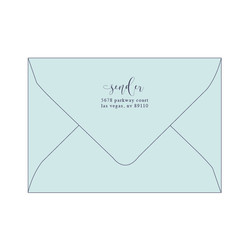s q u a r ef a n c yo b l o n gd i g i t a lc l e a rr s v ph e a r tr i b b o n ( f r o n t )d i g i t a lr i b b o n ( b a c k )d i g i t a lr e c t a n g l ec i r c l eo v a ld i g i t al